## How to solve indefinite integral of x minus 2 divided by x square minus 4x plus 3 by dx ?

Short answer: indefinite integral of x minus 2 divided by x square minus 4x plus 3 by dx is (1/2)ln|x^2-4x+3|)+C. ∫ ((x-2)/(x^2-4x+3))dx is not particulary hard integral. You can solve it in 6 easy steps. We will walk you through and explain everything. Let's start.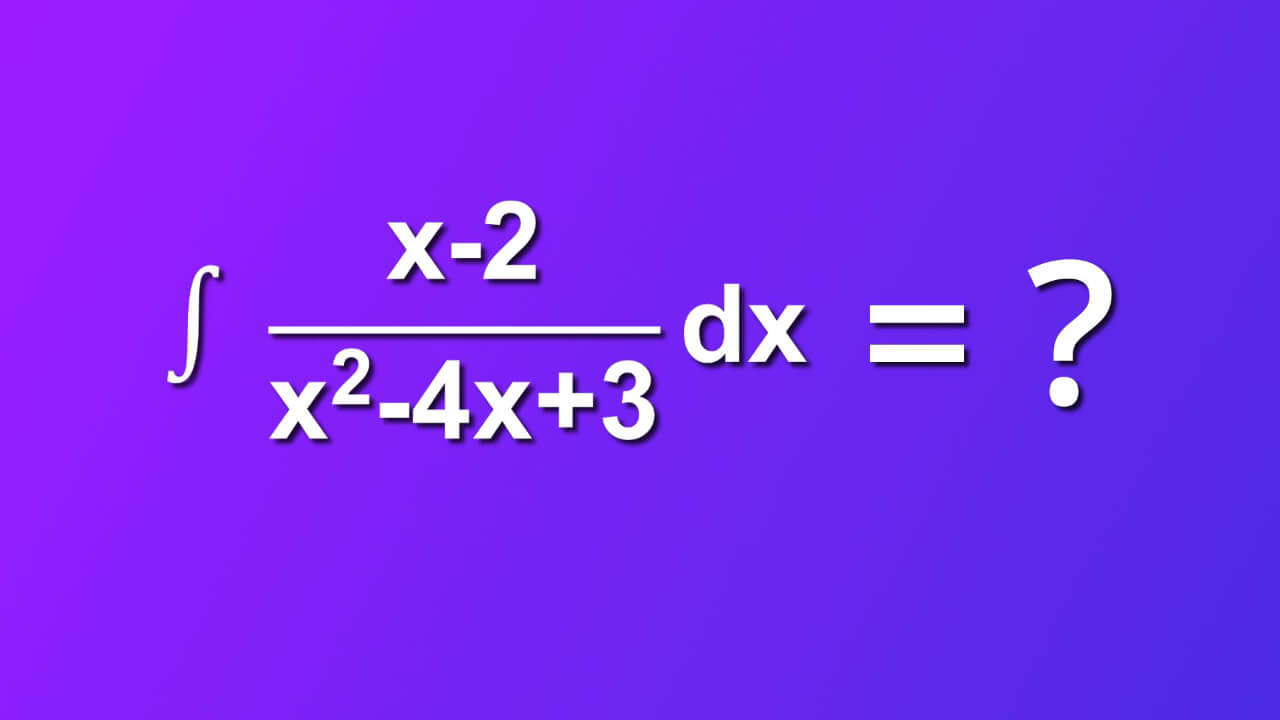## Required assumtions

Usually, we have some additional info about function f of (x). In our case:

1. f(x) belongs to real numbers
2. f(x) is integrable in that domain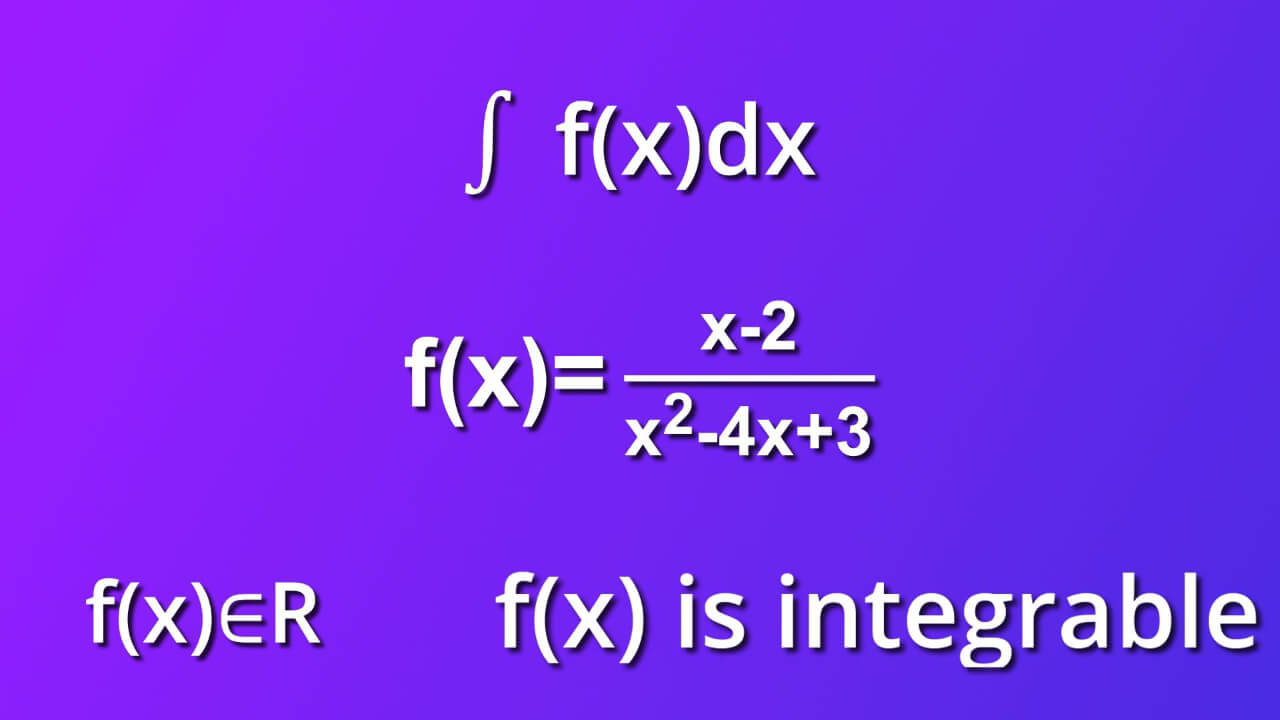## Step by step solution of ∫ ((x-2)/(x^2-4x+3))dx

We will solve ∫ ((x-2)/(x^2-4x+3))dx in 6 easy steps. Let's get started

### Step 1

From symbol dx we know that differential of variable x indicates that the variable of integration is x. In that case, to solve this integral we may apply so called u−substitution method. We do it in order to modify the integral in the way that enable using some of formulas for finding integrals. Now, we try to substitute expression x square minus 4x plus 3.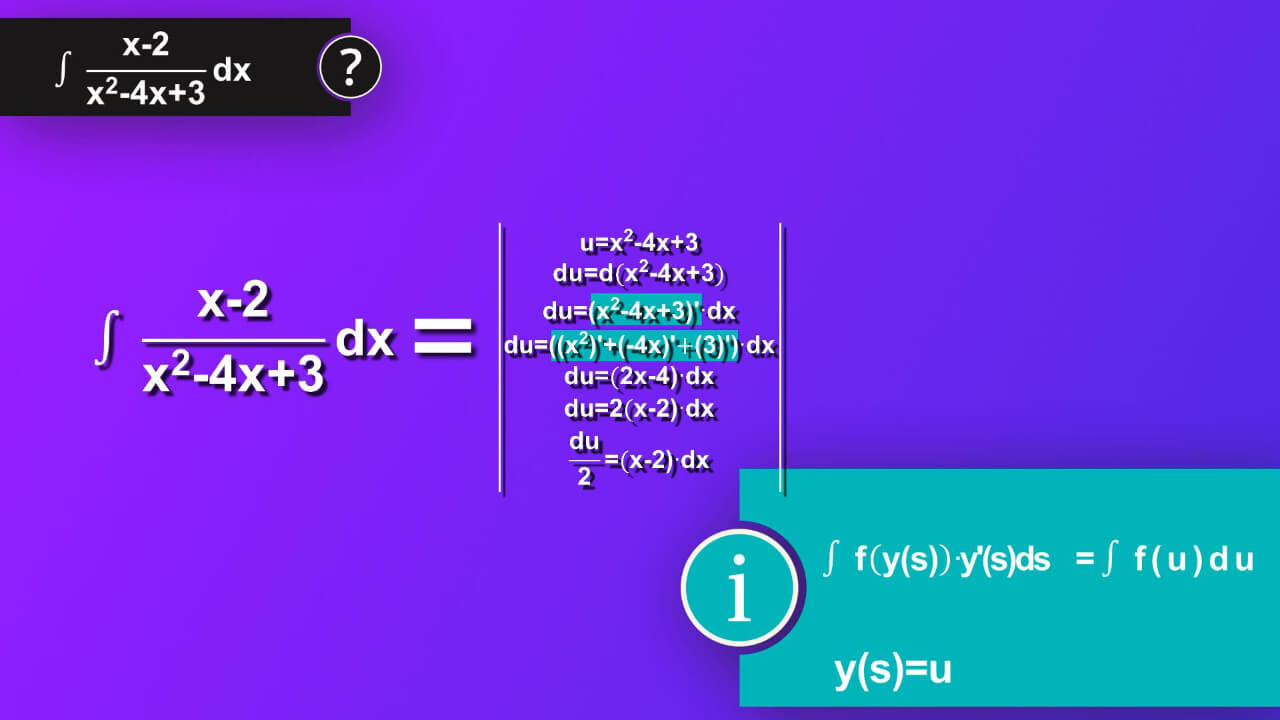### Step 2

We say u is equal to x square minus 4x plus 3. We also have to know what the derivative of u is. So we write derivative of u equals d (x square minus 4x plus 3). So we’ve got du is equal to derivative of x square minus 4x plus 3 multiplied by dx. From rules that we can apply to derivatives, we know that derivative of x square minus 4x plus 3 equals derivative of x square plus derivative of -4x plus derivative of 3 and the whole expression is multiplied by dx. Thus, we have du equals 2 x square as derivative of s to n equals n multiply by s rise to n-1 and in our case s is x and n is 2. Next, we can apply the same formula but now s is x and n is 1 and we’ve got minus 4.From expression 3 we’ ve got zero as derivative of constant is 0. We can write du is equal to 2x minus 4 multiplied by dx. We can write 2 before bracket and finally we will have du divided by 2 is equal to x minus 2 multiplied by dx. In that way we have exact expression in numerator of our main equation.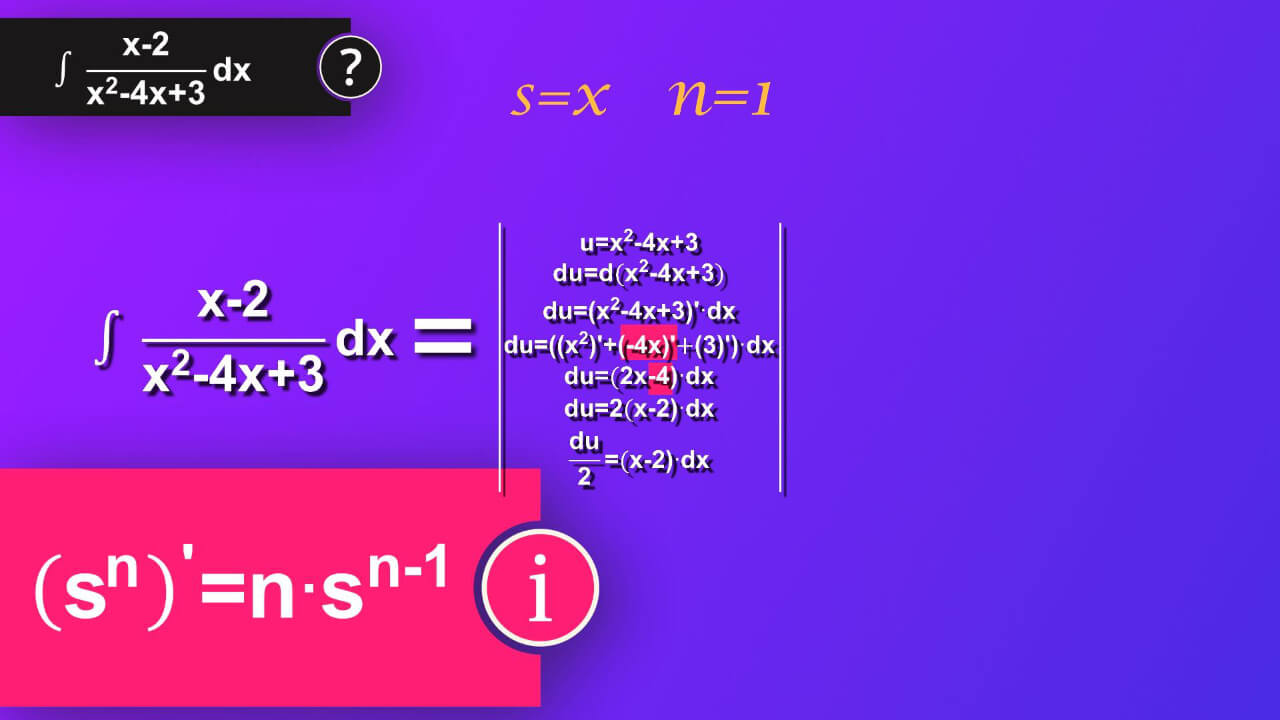### Step 3

Let’s make use of our u. We insert u instead of x square minus 4x plus 3 to our main equation and instead of the whole numerator multiplied by dx we can now insert du divided by 2. Thus, we have integral of du divided by 2 in numerator and u in denominator.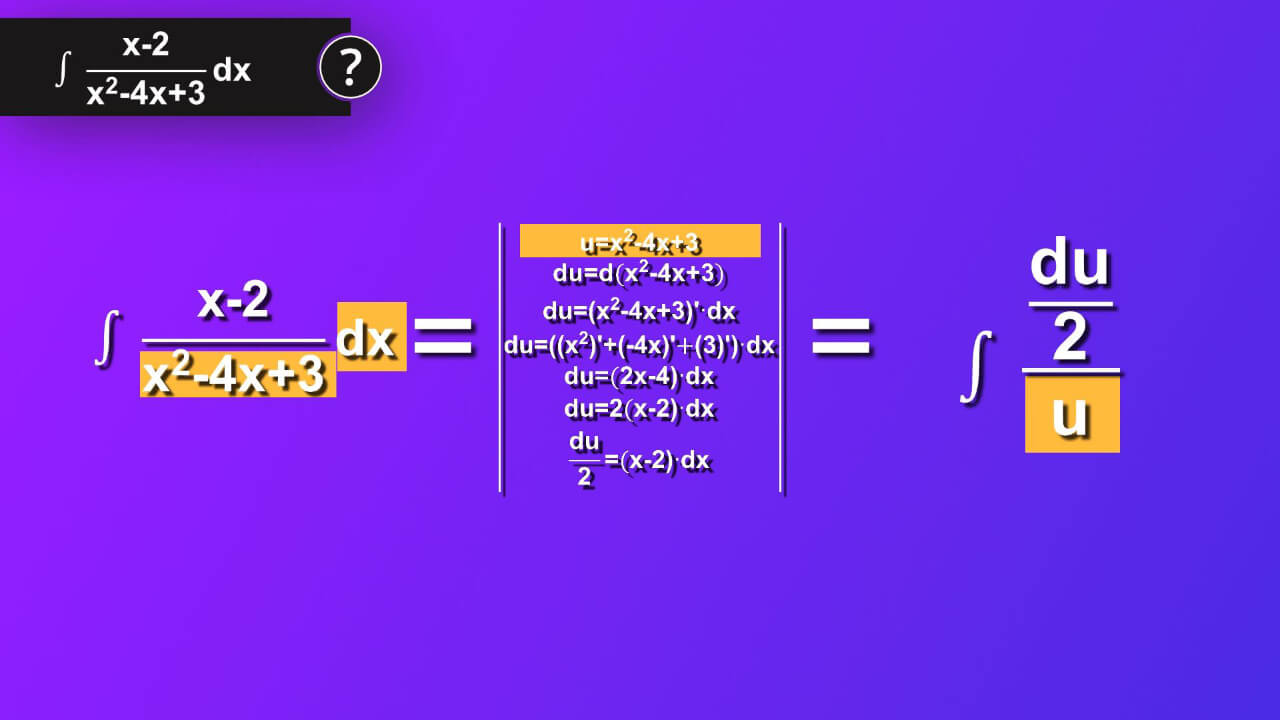### Step 4

We can rewrite this expression as integral of a half multiplied by 1 divided by u multiplied by du. Let’s apply formula which says integral of constant a multiplied by variable s by ds equals constant a multiplied by integral of variable s by ds. In our case s is 1 divided by u and a is a half. We’ve got a half multiplied by integral of 1 divided by u by du.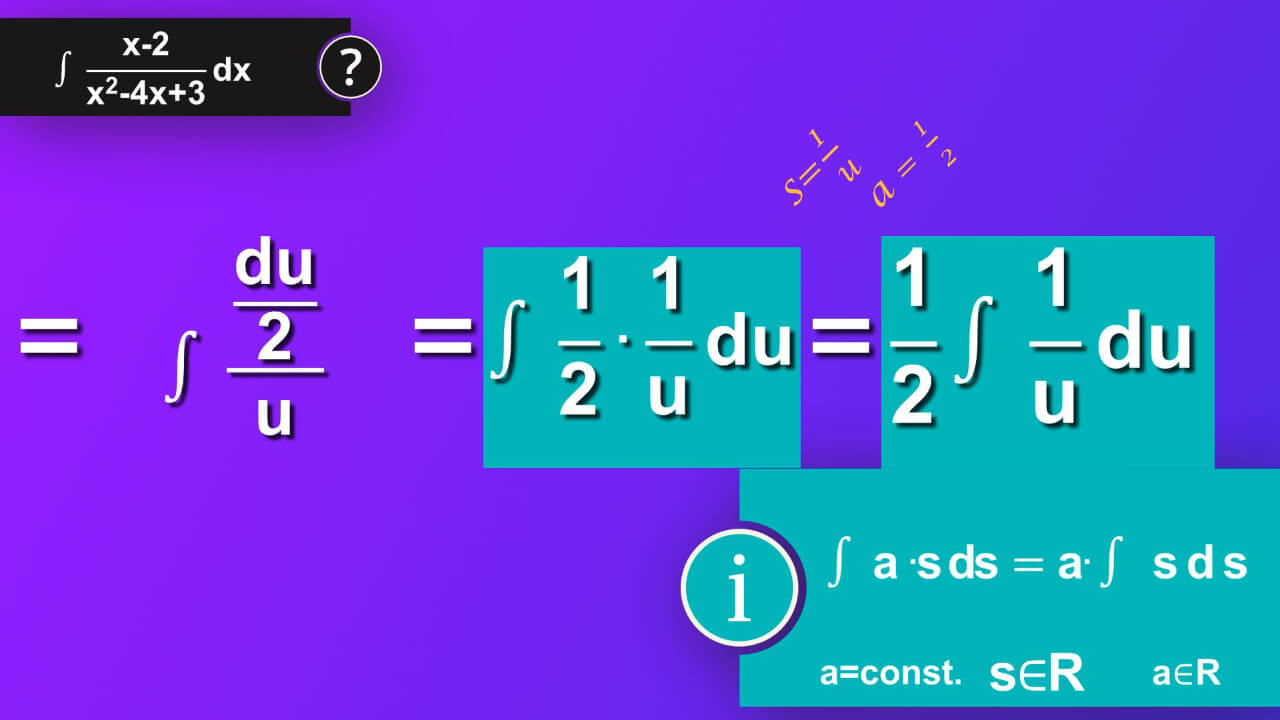### Step 5

Perfect, now we can apply formula for solving integral of one divided by s by ds which equals natural logarithm of absolute value of s. We also must add constant C to make sure that we have all our solution taken into account as our solution make whole class of function not a single one. In our case s is equal to u.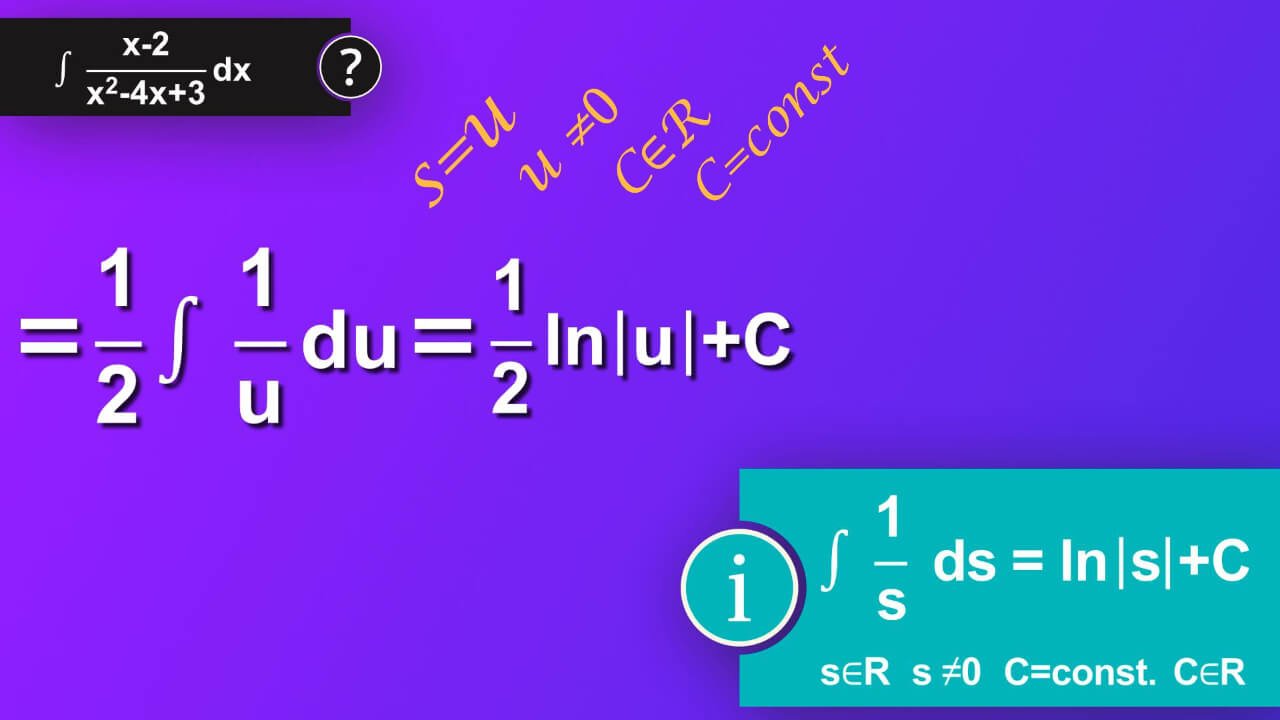### Step 6

Then, we’ve got a half multiplied by natural logarithm of absolute value of u plus constant C. But it is not the end of our solution. We must come back to our problem, and it was formulated using variable x. We used u-substitution and now we must come back to x. So instead of u we now insert x square minus 4x plus 3. We’ve got a half multiplied by natural logarithm of absolute value of (x square minus 4x plus 3) plus constant C as a result of our problem.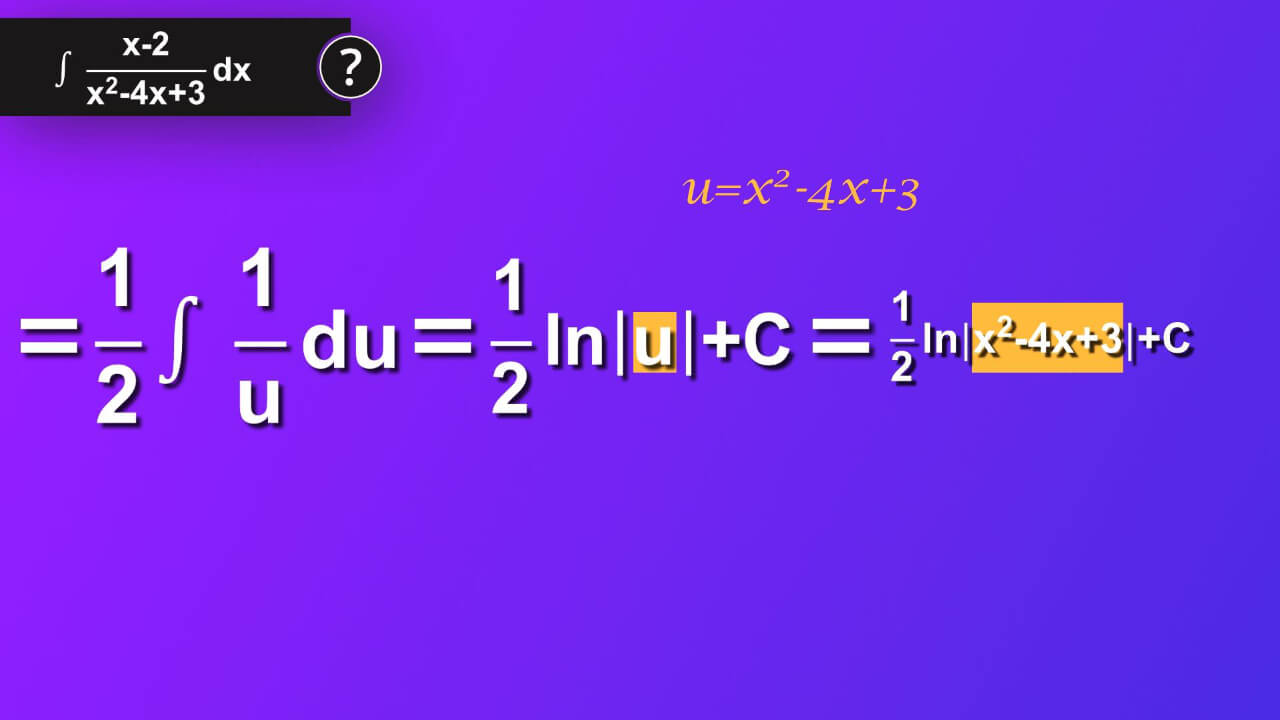## What is indefinite integral of x minus 2 divided by x square minus 4x plus 3 by dx?

We finally did it: ∫ ((x-2)/(x^2-4x+3))dx=(1/2)ln|x^2-4x+3|)+C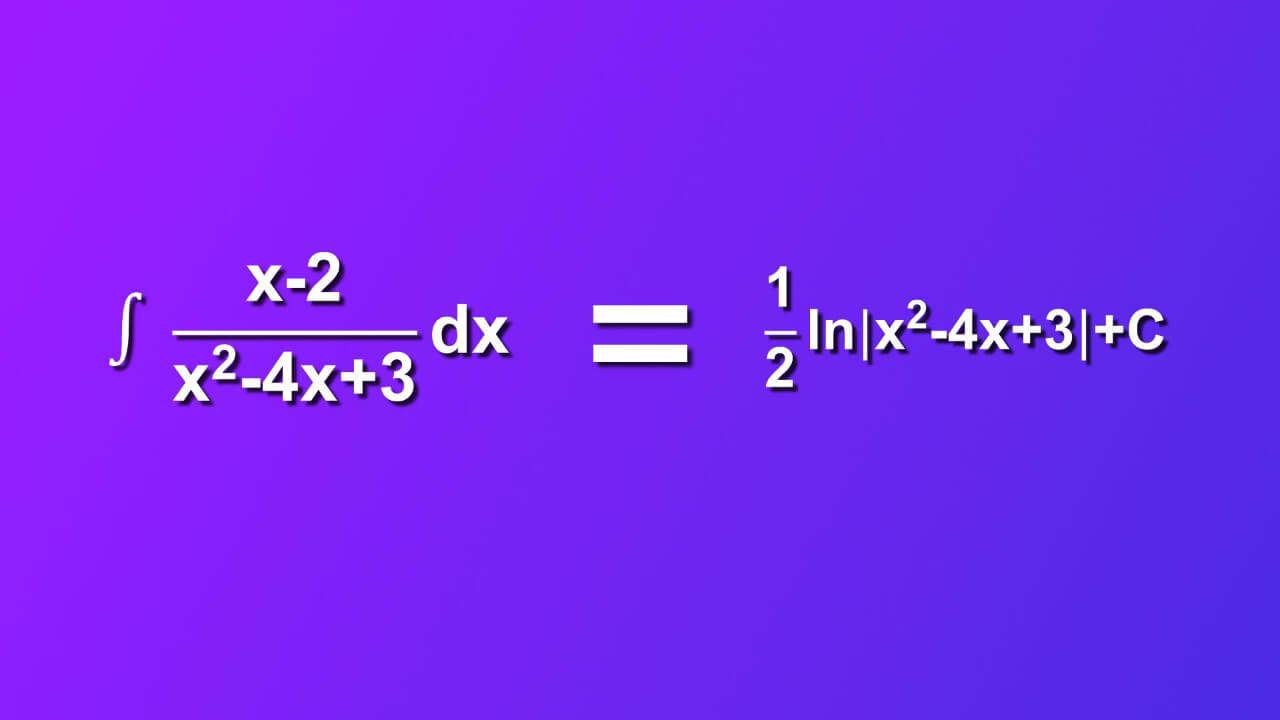## Dictionary

### Integration (antidifferentiation)

Computation (process of finding) of an integral, opposite process to differentiation.

### Integrand

Function placed between sign of integral and differential of variable of integration e.g. $${{ \int f(x)dx}}$$,
where:
$${{ \int }}$$- integration operator,
f(x) – integrand,
dx- differential of variable of integration x

### Integrable function

Function that integral over its domain is finite.

### Indefinite integral

Represents a class of primitive functions whose derivative is the integrand e.g. $${{\int f(x)dx=F(x)+C \Leftrightarrow F’(x)=f(x)}}$$
$${{C=const. }}$$,
$${{f, F,C \in R }}$$
R-real numbers
$${{\int f(x)dx}}$$ - indefinite integral of function f(x) by dx,
$${{F(x)+C}}$$ – a class of primitive functions that $${{F’(x)=f(x) }}$$,
F(x) - primitive function, usually written in capital letters,
R-real numbers.

### Integration by substitution, substitution method, u-substitution

Method used to solve integral when an integral contains some function and its derivative. The function we sat as equal to u and we rewrite the integral using a new variable u.
$${{\varphi: x \rightarrow \varphi (x)=u, x \in X, u \in U}}$$
and
$${{f: u \rightarrow f(u), u \in U, f(u) \in \varphi }}$$
$${{\varphi}}$$ is injective and differentiable function in X
f is continuous in U
then, $${{ \int f(\varphi (x)) \varphi’(x)dx=f(u) du}}$$ $${{\int f(\varphi (x))\varphi’(x)dx=\Bigg|\matrix{u= \varphi (x) \cr d(u)= d(\varphi (x))\cr du= \varphi ’(x) dx}\Bigg| =\int f(u) du}}$$

### Function

Function specified on a set X and having values in set Y is an assignment each element of set X specifically one element in set Y.
$${{f: X \rightarrow Y}}$$
f-function name,
X-set of elements of function f, domain of a function f
Y-set of function values of function f, codomain of a function f
$${{x\in X, y \in Y}}$$
$${{y=f(x) f: x\rightarrow y}}$$
$${{y=y(x), }}$$
y(x)-vales of the function named y,
x-independent variable,
y-dependent variable.

### Composition of functions

Function f(x) and g(x) are specified on set X,
$${{h: X\rightarrow T}}$$ and h(x)=t for x\inX and t\inT
$${{g: T\rightarrow Y}}$$
$${{x\in X, t\inT, y\in Y}}$$
$${{x \buildrel h\over\longrightarrow h(x) \buildrel g\over\longrightarrow g(t)=y}}$$
$${{x\rightarrow y=g(t)|_{t=h(x)}=g(h(x)) }}$$
$${{g(h(x)) }}$$ is a composition of two functions $${{ (g \circ h) (x) }}$$
$${{x \buildrel { g \circ h }\over\longrightarrow y=(g \circ h) (x) }}$$
where:
h-internal function,
g-external function.

### Derivative, derivative of a function

If $${{lim {\Delta f \over \Delta x }}}$$exists (and is finite), then this limit is named
$${{ \Delta x\rightarrow 0}}$$
derivative of a function f(x) in a point x0 and use symbol f’(x).
$${{ f’(x_0) {\buildrel\rm def\over=} \lim_{\Delta x\to0} {\Delta f \over \Delta x}}}$$

### Derivative function f’(x)

Derivative function of function f(x) is a function that values in a point x_0 are equal to derivative of this function in the point x_0.
f’(x_0) - derivative of a function f(x) in a point x_0 (a number, because it is a specifically determined limit of a function),
f’(x)-derivative function.

### Differentiable function

f(x) is differentiable in a point x_0 means that there exists f’(x_0 )
-f(x) is differentiable in (a,b) means there exists f’(x), x $${{\in(a,b) }}$$
-f(x) is differentiable in means there exists $${{f’(x), x \in}}$$

### Differential of a function (df)

Differential of a function f(x) in a point x_0 is symbolized as df(x_0) and $${{df(x_0) {\buildrel\rm def\over=} f’(x_0) \Delta x}}$$